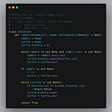# [LeetCode]#1252. Cells with Odd Values in a Matrix

`Input: n = 2, m = 3, indices = [[0,1],[1,1]]Output: 6Explanation: Initial matrix = [[0,0,0],[0,0,0]].After applying first increment it becomes [[1,2,1],[0,1,0]].The final matrix will be [[1,3,1],[1,3,1]] which contains 6 odd numbers.`
`Input: n = 2, m = 2, indices = [[1,1],[0,0]]Output: 0Explanation: Final matrix = [[2,2],[2,2]]. There is no odd number in the final matrix.`
• `1 <= n <= 50`
• `1 <= m <= 50`
• `1 <= indices.length <= 100`
• `0 <= indices[i] < n`
• `0 <= indices[i] < m`
1. Greate m x n matrix.
2. add 1 based on in indices.
3. Sum all old number.
`class Solution:    def oddCells(self, n, m, indices):        count=0        matrix=[*m for i in range(n)]        for c in indices:            x,y=c,c            for i in range(m):                matrix[x][i]+=1            for j in range(n):                matrix[j][y]+=1        for i in range(n):            for j in range(m):                if matrix[i][j] % 2 !=0:                    count+=1        return count`

## More from Fatboy Slim

Interesting in any computer science.

Love podcasts or audiobooks? Learn on the go with our new app.

## Origin: Warranty Tracker## 17 Basic Linux Commands Every Developer should Know## Cloud management done right## Flutter State Management with Provider — NotifyListeners x StreamProvider — Part 1## Promises in JavaScript: Explained for Kids## Google Colab mount Google Drive and connect with Weights and Biases to track model training…## Chocolatey and Gist## Fatboy Slim

Interesting in any computer science.

## [LeetCode]#2053. Kth Distinct String in an Array